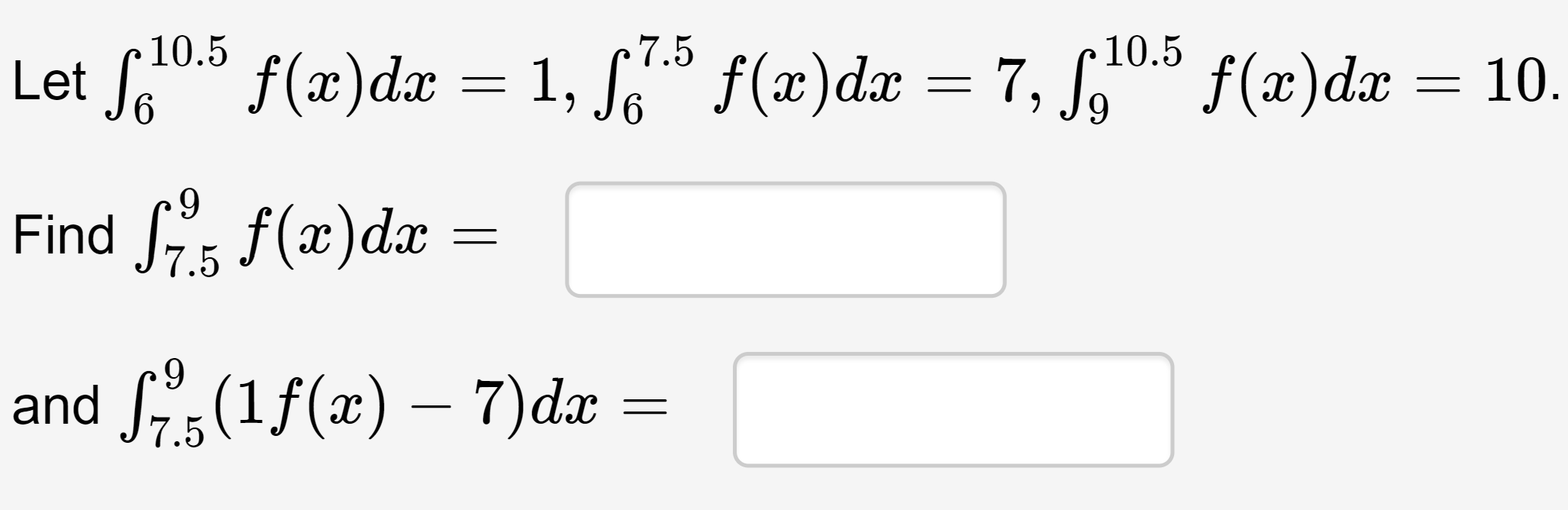# 7.5 10.5 10.5 Let fou = 7, S,0" f(x)dx f(x)dx = 1, S f(x)dx 10. 9. Find , f(x)dx 7.5 and S(1f(x) – 7)da =

Questionhelp_outlineImage Transcriptionclose7.5 10.5 10.5 Let fou = 7, S,0" f(x)dx f(x)dx = 1, S f(x)dx 10. 9. Find , f(x)dx 7.5 and S(1f(x) – 7)da = fullscreen